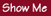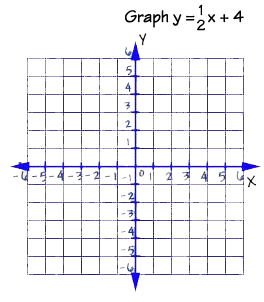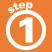Home    |    Teacher    |    Parents    |    Glossary    |    About UsTo graph a linear equation, we can use the slope and y-intercept.

1. Locate the y-intercept on the graph and plot the point.2. From this point, use the slope to find a second point and plot it.3. Draw the line that connects the two points.Homework Help | Algebra | Graphing Equations and InequalitiesEmail this page to a friendSearch·  The coordinate plane·  Slope and y-intercept·  Graphing linear     equationsFirst Glance In Depth Examples WorkoutGraphing linear equations